# Review — A Pattern Classification Based Approach for Blur Classification (Blur Classification)

## Blur Classification Using Curvelet Transform + Neural Network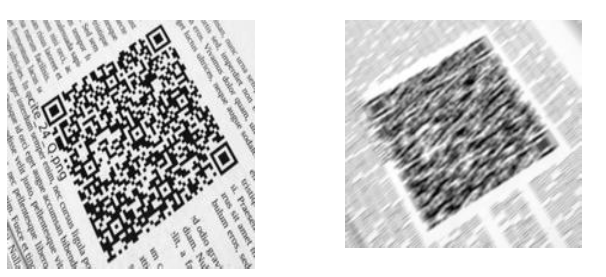Motion Blur of a QR Code

In this story, A Pattern Classification Based Approach for Blur Classification, (Tiwari IJEEI’17), by Mody University of Science & Technology, is reviewed. In this paper:

• Curvelet transform based energy features are utilized as features of blur patterns and a neural network is designed for blur classification: motion, defocus, and combined blur.

This is a paper in 2017 IJEEI. (Sik-Ho Tsang @ Medium)

# Outline

1. Pre-Processing
2. Feature Extraction Using Curvelet Transform
3. Neural Network
4. Experimental Results

# 1. Pre-processing

• First, a color image is converted into an 8-bit grayscale image.
• Then, Hanning window is applied to the image.
• The windowed image is transformed to the frequency domain using Fourier transform.
• A centered portion of size 256×256 is cropped to perform feature extraction.

# 2. Feature Extraction Using Curvelet Transform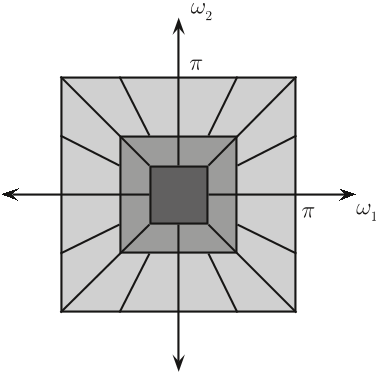Wrapping based discrete curvelet transform with (1, 8, 16)-Orientation at 3-Scale
• Wrapping based discrete curvelet transform using Curvelab-2.1.2 is applied to a power spectrum of barcode image to obtain its coefficients.
• These coefficients are then used to form the features of blur patterns.
• After achieving the curvelet coefficients, the mean and standard deviation of the coefficients related with each subband is calculated at the coarsest and the finest scale independently.
• The mean of a subband at scale j and orientation l is calculated as:
• where M×N is the size and E(j,l) is the energy of curvelet transformed image respectively at scale j and orientation l.
• Energy is calculated by the sum of absolute values of curvelet coefficients: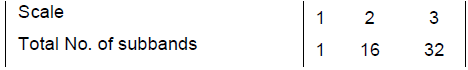Wrapping based discrete curvelet transform with (1, 16, 32)-Orientation at 3-Scale
• In this paper, the above wrapping based discrete curvelet transform, with (1, 16, 32)-Orientation at 3-Scale, is used.
• Only first half of the total subbands at a scale are considered for feature calculation, since the remaining subbands at another side have similar coefficients.
• Thus, (1+8+16) = 25 subbands of curvelet coefficients are selected for calculation of mean and standard deviation.
• Finally, a feature vector with length of 50 is obtained. The standard deviations remain in the first half of the feature vector and the means are arranged into the second half of the feature vector.

# 3. Neural Network

• The whole training and testing features set is normalized into the range of [0, 1].
• Hyperbolic tangent sigmoid functions are used as the activation function.
• The final architecture is selected with single hidden layer of fifty neurons which gives best performance. (Not much details on this.)

# 4. Experimental Results

• Two different barcode image databases are considered.
• The first database WWU Muenster Barcode Database  consisting of 1D barcode images and the second one is the Brno Institute of Technology QR code image database  captured by digital camera.
• The data is divided into training, validation and test sets in a ratio of 50:20:30 respectively.

## 4.1. 1D Barcode Database

• First 200 images from the 1D barcode database are considered.
• Then, the three different classes of blur (motion, defocus and combined blur) were synthetically introduced with different parameters to make the database of 600 blurred images (i.e., 200 images with each class of blur) for each type of barcode images.
• The best validation performance is 0.0115, at epoch 39, as shown above.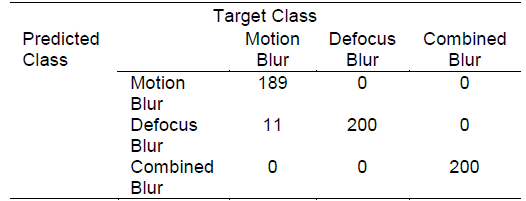Confusion matrix for blur classification results of blurred 1D barcode images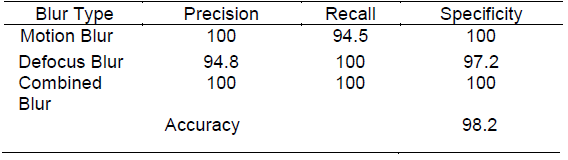Blur Classification results for blurred 1D barcode images
• The overall classification accuracy is 98.2%.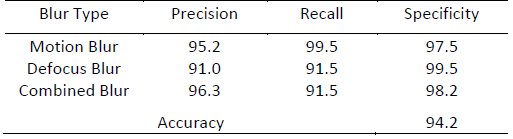Blur Classification results for blurred and noisy 1D barcode images
• Blurred and noisy 1D images are also tested.
• The overall classification accuracy is 94.2%.

## 4.2. QR Code Database

• First 200 images from the QR code database are considered.
• The same procedure is followed to produce 600 blurred images.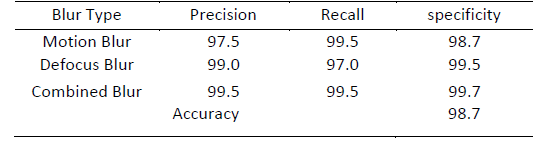Blur Classification results for blurred and noisy 2D barcode images
• The overall classification accuracy is achieved as 98.7%.
• Blurred and noisy QR code images are also tested.
• The overall classification accuracy is 96.3%.

## 4.3. SOTA Comparison

• The proposed method in this paper outperforms wavelet transform plus feed forward neural network (Tiwari IJMECS’14) .

## Reference

[2017 IJEEI] [Tiwari IJEEI’17]
A Pattern Classification Based Approach for Blur Classification

## Blur Classification

2014 [Tiwari IJMECS’14] 2017 [Tiwari IJEEI’17] [SFA] 2019 [SFA & SFGN] 2020 [Szandała SSCI’20] [Tiwari IJISMD’20]

--

--